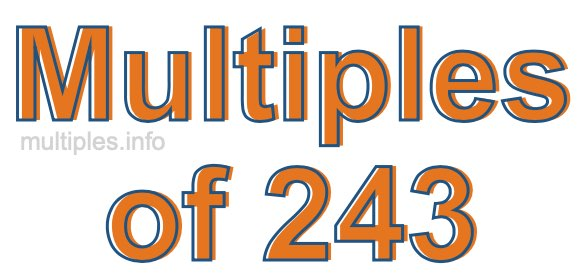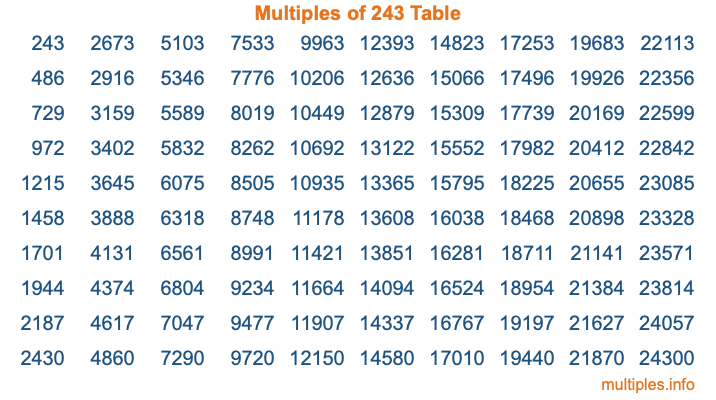Multiples of 243Welcome to the Multiples of 243 page. Here we will first teach you everything you will ever need to know about the multiples of 243, and then give you a study guide summary of everything we taught you to make sure you remember it all. Use this page to look up facts and learn information about the multiples of 243. This page will make you a multiples of two hundred forty-three expert!

Definition of Multiples of 243
Multiples of 243 are all the numbers that when divided by 243 equal an integer. Each of the multiples of 243 are called a multiple. A multiple of 243 is created by multiplying 243 by an integer.

Therefore, to create a list of multiples of 243, you start with 1 multiplied by 243, then 2 multiplied by 243, then 3 multiplied by 243, and so on for as long as you want. Thus, the list of the first five multiples of 243 is 243, 486, 729, 972, and 1215. To see a larger list of multiples of 243, see the printable image of Multiples of 243 further down on this page. We also have a category where you can choose any nth multiple of 243.

Multiples of 243 Checker
The Multiples of 243 Checker below checks to see if any number of your choice is a multiple of 243. In other words, it checks to see if there is any number (integer) that when multiplied by 243 will equal your number. To do that, we divide your number by 243. If the the quotient is an integer, then your number is a multiple of 243.

Is  a multiple of 243?

Least Common Multiple of 243 and ...
A Least Common Multiple (LCM) is the lowest multiple that two or more numbers have in common. This is also called the smallest common multiple or lowest common multiple and is useful to know when you are adding our subtracting fractions. Enter one or more numbers below (243 is already entered) to find the LCM.

Check out our LCM Calculator if you need more details about the Least Common Multiple or if you need the LCM for different numbers for adding and subtraction fractions.

nth Multiple of 243
As we stated above, 243 is the first multiple of 243, 486 is the second multiple of 243, 729 is the third multiple of 243, and so on. Enter a number below to find the nth multiple of 243.

th multiple of 243

Multiples of 243 vs Factors of 243
243 is a multiple of 243 and a factor of 243, but that is where the similarities end. All postive multiples of 243 are 243 or greater than 243. All positive factors of 243 are 243 or less than 243.

Below is the beginning list of multiples of 243 and the factors of 243 so you can compare:

Multiples of 243: 243, 486, 729, 972, 1215, etc.

Factors of 243: 1, 3, 9, 27, 81, 243

As you can see, the multiples of 243 are all the numbers that you can divide by 243 to get a whole number. The factors of 243, on the other hand, are all the whole numbers that you can multiply by another whole number to get 243.

It's also interesting to note that if a number (x) is a factor of 243, then 243 will also be a multiple of that number (x).

Multiples of 243 vs Divisors of 243
The divisors of 243 are all the integers that 243 can be divided by evenly. Below is a list of the divisors of 243.

Divisors of 243: 1, 3, 9, 27, 81, 243

The interesting thing to note here is that if you take any multiple of 243 and divide it by a divisor of 243, you will see that the quotient is an integer.

Multiples of 243 Table
Below is an image of the first 100 multiples of 243 in a table. The table is in chronological order, column by column. The first column has the first ten multiples of 243, the second column has the next ten multiples of 243, and so on.The Multiples of 243 Table is also referred to as the 243 Times Table or Times Table of 243. You are welcome to print out our table for your studies.

Negative Multiples of 243
Although not often discussed or needed in math, it is worth mentioning that you can make a list of negative multiples of 243 by multiplying 243 by -1, then by -2, then by -3, and so on, to get the following list of negative multiples of 243:

-243, -486, -729, -972, -1215, etc.

Multiples of 243 Summary
Below is a summary of important Multiples of 243 facts that we have discussed on this page. To retain the knowledge on this page, we recommend that you read through the summary and explain to yourself or a study partner why they hold true.

There are an infinite number of multiples of 243.

A multiple of 243 divided by 243 will equal a whole number.

243 divided by a factor of 243 equals a divisor of 243.

The nth multiple of 243 is n times 243.

The largest factor of 243 is equal to the first positive multiple of 243.

243 is a multiple of every factor of 243.

243 is a multiple of 243.

A multiple of 243 divided by a divisor of 243 equals an integer.

243 divided by a divisor of 243 equals a factor of 243.

Any integer times 243 will equal a multiple of 243.

Multiples of a Number
Here you can get the multiples of another number, all with the same attention to detail as we did for multiples of 243 on this page.

Multiples of
Multiples of 244
Did you find our page about multiples of two hundred forty-three educational? Do you want more knowledge? Check out the multiples of the next number on our list!